# Inductance

By on

When current flows within a wire, a magnetic field is created. The potion of this magnetic field perpendicular to the wire is called the magnetic flux (measured in weber, Wb). Inductance is the ratio of magnetic flux to current in a circuit. The unit of inductance is the henry, H (Wb/A) and is normally represented by the symbol L.

## Self Inductance

Whenever current in a coil of wire changes,  the magnetic field]it produces will change. That will change the magnetic flux through the coil and hence produce a voltage across the coil. This is called self-inductance, and the coil is referred to as an inductor.

### Example – Self Inductance of a Solenoid

Consider a coil of wire around a magnetic core, whose length is much greater than it's diameter.

Given the permeability of free space μo , relative permeability of the magnetic core μr , number of turns N and length of solenoid l, with a current i flowing, the magnetic flux density, B within the coil is is given by: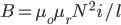The magnetic flux, Φ is obtained by multiplying flux density by the cross sectional area A: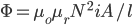Given that the inductance is the ratio of magnetic flux to current, we have: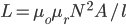Note: if the solenoid is wound around a non-magnetic core, then μr = 1.

## Mutual Inductance

When the magnetic field of one coil links with that of another coil, a change in current in the first coil will produce a linking magnetic field with the second coil.  The magnetic field linking with the second coil will produce a voltage within that coil.  This is called mutual inductance.

For two inductors (coils) we have:

• L11 – self inductance of inductor 1
• L22 – self inductance of inductor 2
• L12 = L21 – mutual inductance between the two

## Inductance Formulae

The table below lists some common formulae for calculating the theoretical inductance of several inductor constructions.

• L = inductance (H)
• μ0 = permeability of free space = 4 π× 10-7 (H/m)
• μr = relative permeability of core material

Cylindrical coil: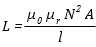N = number of turns A = area of cross-section of the coil in square metres (m2) l = length of coil in metres (m)

Straight wire conductor: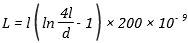l = length of conductor (m) d = diameter of conductor (m)

Flat spiral air-core coil: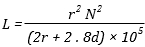r = mean radius of coil (m) N = number of turns d = depth of coil (outer radius minus inner radius) (m)

Toroidal core, circular cross-section: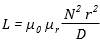N = number of turns r = radius of coil winding (m) D = overall diameter of toroid (m)

## Application to Circuits

The quantitative measure of applying an inductance L, to a circuit defined by: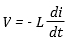An inductor can store energy. The power (= energy / time) being stored in an inductor is: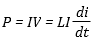This implies (by a little calculus) that the energy stored in an inductor is :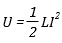More interesting Notes:Steven McFadyen

Steven has over twenty five years experience working on some of the largest construction projects. He has a deep technical understanding of electrical engineering and is keen to share this knowledge. About the author

#### View 2 Comments (old system)

1.Chris says:
7/12/2012 3:52 AM

You list the relative permeability of non-magnetic materials as 0. Shouldn't it be 1?

•Steven says:
7/14/2012 9:45 AM

Thanks for spotting the error Chris. I have corrected it.

Comments are closed for this post:
• have a question or need help, please use our Questions Section
• spotted an error or have additional info that you think should be in this post, feel free to Contact Us

UPS - Uninterruptible Power Supply

A UPS is an uninterruptible power supply.  It is a device which maintains a continuous supply of electrical power, even in the event of failure of the...

Earth Electrode Resistance

Earthing of electrical systems is essential for the correct functioning and the protecting of life and equipment in the event of faults.  The earth electrode...

Standard Cable & Wire Sizes

IEC 60228 is the International Electrotechnical Commission's international standard on conductors of insulated cables. Among other things, it defines a...

Switchboard - Forms of Internal Separation

IEC 61439 'Low-voltage switchgear and controlgear assemblies', specifies standard arrangements of switchboard (call forms of internal separation). The...

Three phase systems are derived from three separate windings, either connected in delta or star (wye). Each winding can be treated separately, leading...

How to Check a Circuit is Dead

If you want to check a circuit is dead (not live), you should always use the three point method. First check a known live circuit, then check the dead...

Railway Electrification Voltages

This post is quick introduction and overview to different railway electrification voltages used in answer to a question sent in via email. While there...

Induction Motor Calculator

Just added a page to the tools, which will allow you to calculate the synchronous speed, slip and rated torque for an induction motor. Not a particularly...

Motor Efficiency Classification

Electric motors are one of the most widely used items of electrical equipment. Improving motor efficiency benefits include, reduced power demand, lower...

Back to Basics - Ohm’s Law

Electrical engineering has a multitude of laws and theorems. It is fair to say the Ohm's Law is one of the more widely known; it not the most known. Developed...## Have some knowledge to share

If you have some expert knowledge or experience, why not consider sharing this with our community.

By writing an electrical note, you will be educating our users and at the same time promoting your expertise within the engineering community.

To get started and understand our policy, you can read our How to Write an Electrical Note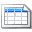Click here to view the notes list in a table formatClick here to view the notes indexed by tag